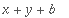Advice: Local procedures don't access local variables

A procedure can define other procedures inside it (using proc or -> ), but these are quite different from local procedures in most programming languages. In particular, they do not have access to the local variables and formal parameters of the first procedure. For example:

> f:= proc(x) local y, g;
y:= a;
g:= t -> x + y + t;
g(b);
end;

> f(c);The x and y in the definition of g are the global variables x and y , not the formal parameter x or local variable y of f .

You can, however, use subs to define a procedure that uses the values of the formal parameters and local variables:

> f:= proc(x) local y, g;
y:= a;
g:= subs(_X=x, _Y=y, (t -> _X + _Y + t));
g(b);
end;

> f(c);This page does not apply to Release 5, in which Maple has lexical scoping.